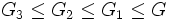# Commutator of finite nilpotent group with coprime automorphism group equals second commutator

## Statement

Let$G$ be a Finite nilpotent group (?) and$A$ be a subgroup of$\operatorname{Aut}(G)$ whose order is relatively prime to the order of$G$. Then, we have:$[[G,A],A] = [G,A]$,

where:$[G,A] = \langle g\sigma(g)^{-1} \mid g \in G, \sigma \in A \}$.

## Facts used

1. Centralizer-commutator product decomposition for finite nilpotent groups: This states that under the same conditions ($G$ a finite nilpotent group and$A$ a subgroup of$\operatorname{Aut}(G)$ of order coprime to the order of$G$), we have$G = [G,A]C_G(A)$, and further, if$K$ is an$A$-invariant subgroup of$G$ such that$G = KC_G(A)$, then$[G,A] \le K$.
2. Nilpotence is subgroup-closed
3. Lagrange's theorem
4. Order of quotient group divides order of group

## Proof

Given: A finite nilpotent group, a subgroup$A$ of$\operatorname{Aut}(G)$ such that the orders of$A$ and$G$ are relatively prime.

To prove:$[[G,A],A] = [G,A]$.

Proof: Let$G_1 = [G,A], G_2 = [G_1,A], G_3 = [G_2,A]$.

1.$G_3 \le G_2 \le G_1 \le G$: Since$G_1 \le G$, we have$[G_1,A] \le [G,A]$, so$G_2 \le G_1$. Thus,$[G_2,A]\le [G_1,A]$, so$G_3 \le G_2$. Thus,$G_3 \le G_2 \le G_1 \le G$.
2.$G = G_1C_G(A)$: This follows directly from fact (1).
3.$G_1 = G_2C_{G_1}(A)$: This follows by applying fact (1), replacing$G$ by$G_1$, and observing that:
• Since$G$ is nilpotent, fact (2) tells us that$G_1$ is nilpotent.
• Since$G$ has order relatively prime to that of$A$, the order of$G_1$ is also relatively prime to$A$ (using fact (3)). Further, since$G_1$ is$A$-invariant, there is a homomorphism$A \to \operatorname{Aut}(G_1)$, with image$A_1$ of order dividing the order of$A$, and so that the action of$A$ on$G_1$ factors through this homomorphism. The order of$A_1$ divides the order of$A$ by fact (4), so we get the conditions for fact (1), yielding$G_1 = [G_1,A_1]C_{G_1}(A_1)$. Finally, since the action of$A$ factors via the homomorphism, we get$G_1 = [G_1,A]C_{G_1}(A) = G_2C_{G_1}(A)$.
4.$G = G_2C_G(A)$: By the two preceding steps,$G = G_2C_{G_1}(A)C_G(A)$. But$C_{G_1}(A) \le C_G(A)$, so we get$G = G_2C_G(A)$.
5.$G_2$ is$A$-invariant: Since$[G_2,A] = G_3 \le G_2$, we in particular have that$G_2$ is$A$invariant.
6.$G_1 \le G_2$: By the preceding two steps,$G_2$ is$A$-invariant and$G = G_2C_G(A)$, so by fact (1), we have$G_1 \le G_2$.
7.$G_1 = G_2$: This follows from the preceding step, combined with step (1).